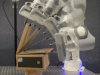### Abstract

Daily manipulation tasks are characterized by regular characteristics associated with the task structure, which can be described by multiple geometric primitives related to actions and object shapes. Such geometric descriptors can not be expressed only in Cartesian coordinate systems. In this paper, we propose a learning approach to extract the optimal representation from a dictionary of coordinate systems to represent an observed movement. This is achieved by using an extension of Gaussian distributions on Riemannian manifolds, which is used to analyse a set of user demonstrations statistically, by considering multiple geometries as candidate representations of the task. We formulate the reproduction problem as a general optimal control problem based on an iterative linear quadratic regulator (iLQR), where the Gaussian distribution in the extracted coordinate systems are used to define the cost function. We apply our approach to grasping and box opening tasks in simulation and on a 7-axis Franka Emika robot. The results show that the robot can exploit several geometries to execute the manipulation task and generalize it to new situations, by maintaining the invariant features of the skill in the coordinate system(s) of interest.

### Bibtex reference

@inproceedings{Ti22,
author="Ti, B. and Gao, Y. and Zhao, J. and Calinon, S.",
title="Imitation of Manipulation Skills Using Multiple Geometries",
booktitle="Proc.\ {IEEE/RSJ} Intl Conf.\ on Intelligent Robots and Systems ({IROS})",
year="2022",
pages="",
}